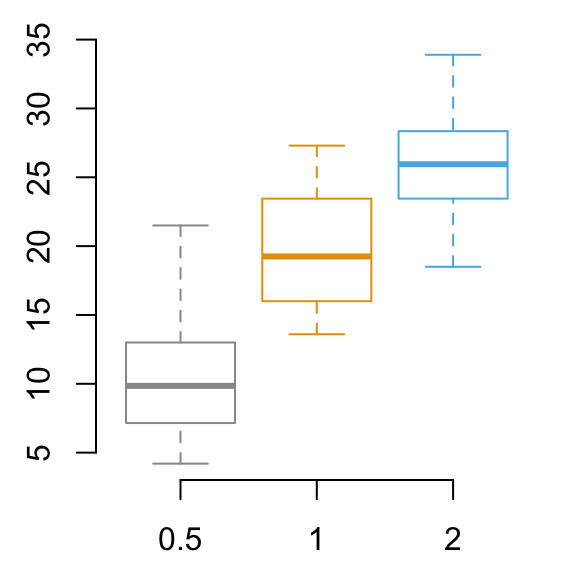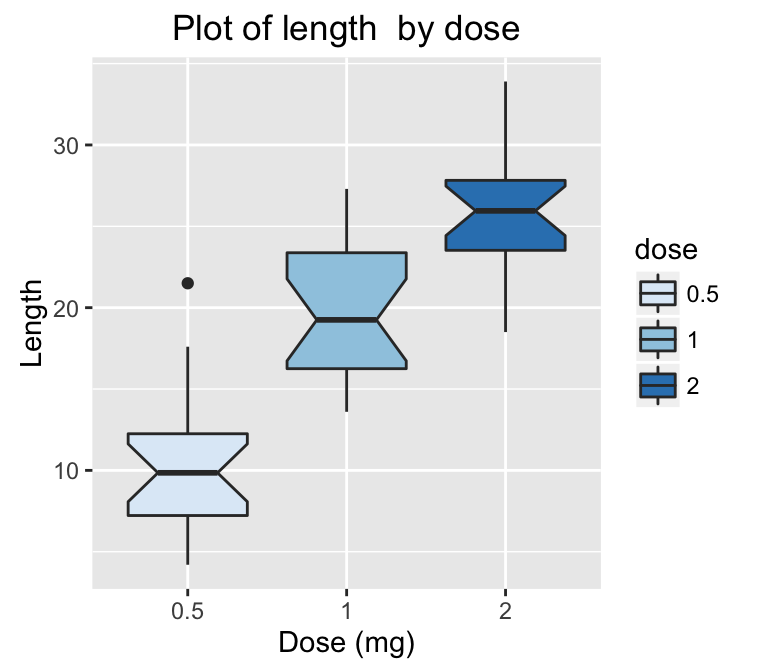# Data Visualization

Previously, we described the essentials of R programming and provided quick start guides for importing data into R.

This chapter describes how to plot data in R and make elegant data visualization.

# R Base GraphsRead more: —> R base Graphs.

# Lattice Graphs

Lattice package is a powerful and elegant data visualization system that aims to improve on base R graphs.• xyplot(): Scatter plot
• cloud(): 3D scatter plot
• Box plot, Dot plot, Strip plot
• Density plot and Histogram

# Ggplot2

ggplot2 is a powerful and a flexible R package, implemented by Hadley Wickham, for producing elegant graphics.# 3D Graphics3d scatter plot rgl

1. Static 3D Scatter Plots
2. Interactive 3D Graphs

# Infos

This analysis has been performed using R statistical software (ver. 3.2.4).